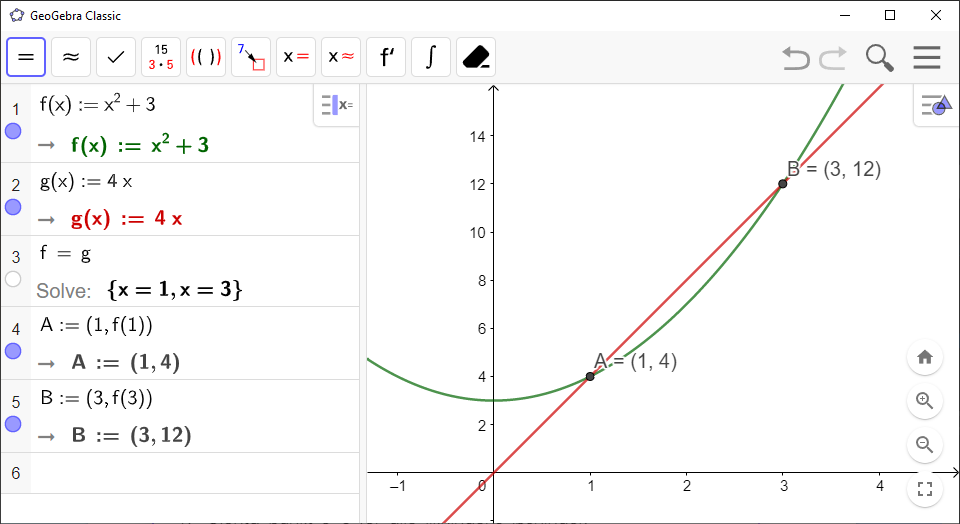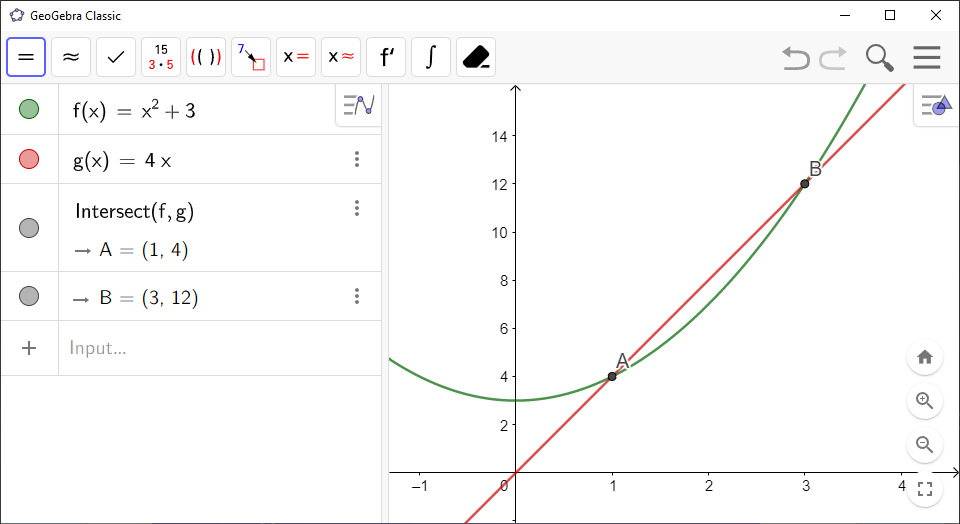Math Topics

# How to Find Intersection Between Graphs in GeoGebra

You can either use `CAS`, or `Algebra View` and `Graphics View` together, to find the points of intersection between graphs. Instructions for both methods are detailed below.

`GeoGebra` Instruction 1

### Using`CAS`

1.
Open `CAS` under`View` in`Menu`.
2.
Type $f\left(x\right)$ in `CAS` as

f(x) := $\dots$

and press `Enter`. Do the same with $g\left(x\right)$.

3.
In an empty row in `CAS`, type

f = g

and click `Solve`in `Toolbar`. You should get a list of solutions.

4.
For each of the solutions `x` in the list from Step 3, type

(x,f(x))

in an empty row in `CAS` and press `Enter`. `GeoGebra` then displays the points of intersection.`GeoGebra` Instruction 2

### Using`Algebra View`and`Graphics View`

1.
Open `Algebra View` and `Graphics View` under`View` in`Menu`.
2.
Type $f\left(x\right)$ into `Algebra View` and press `Enter`. Do the same with $g\left(x\right)$. These now appear as functions in `Algebra View` and as graphs in `Graphics View`.
3.
In `Toolbar`, click `Intersect`(it is under the same menu as the `Point`tool).
4.
Click both graphs. The order does not matter.
5.
You will now find the points of intersection displayed in `Algebra View`.If you wish, you can additionally choose the axes to find the intersection between a graph and the axes.